# Introduction to Optics - Laws of Reflection, Refraction, Plane Mirrors

Everyday you get ready for your school and when you come in front of a mirror you see a person similar to you standing just behind the mirror at the same distance as you are.This can be understood by knowing the optical phenomenons. The mirror in front of you is a plane mirror and the person behind the mirror is the virtual image of yours!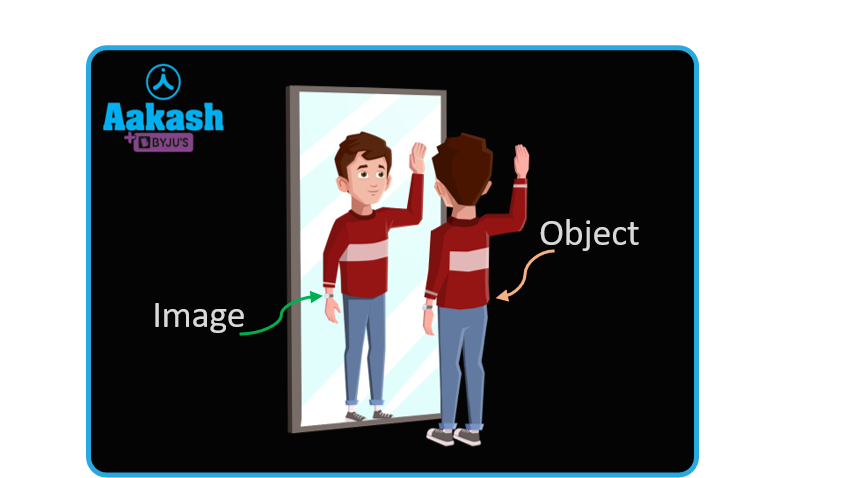## Introduction

Optics or geometric optics or ray optics is an optical model that describes the propagation of light in terms of rays. In ray optics, the propagation of light is represented by a ray, and an arrow represents the direction of the propagation. Therefore, it can be said that in the absence of an obstacle, the rays travel in straight lines without changing directions. The straight line motion of the light is also known as the rectilinear propagation of light. And when this light falls on objects,  images are formed. Let’s see the types of objects.

## Types of Objects

• Luminous objects: The objects that emit light or the objects that produce the light by themselves are known as the luminous objects. Examples: Sun, bulb, candle etc.
• Non-luminous objects: The objects that do not produce their own light are known as the non-luminous objects. Examples: table, chair etc.

## Light

Light is an electromagnetic wave that carries energy and momentum. On the basis of wavelength (or frequency), light can be divided into a spectrum (as shown in the figure) and the spectrum is sometimes known as electromagnetic spectrum.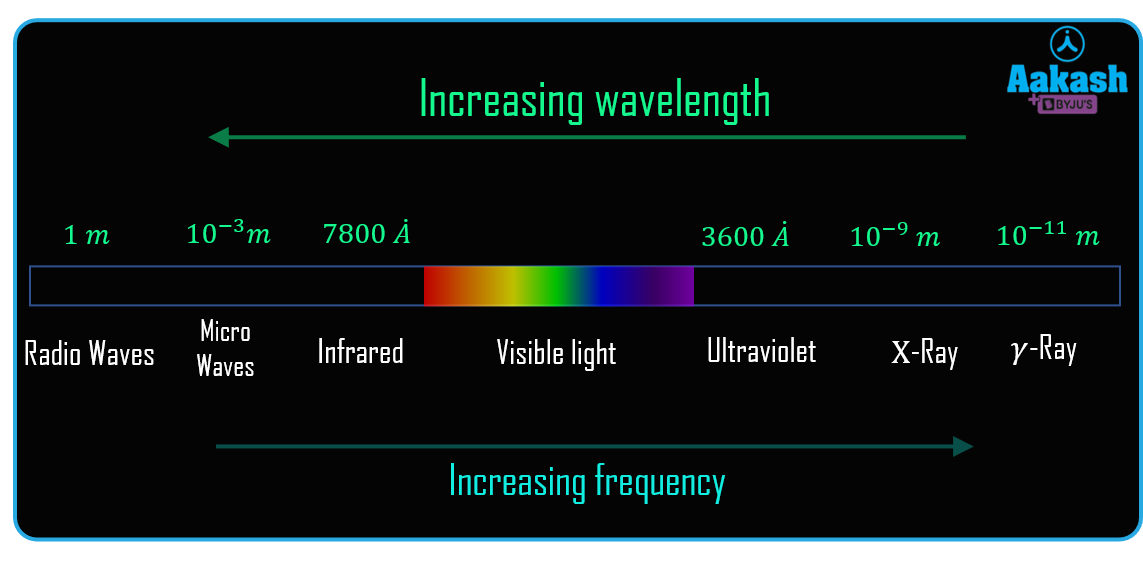Since; higher the frequency, lower will be the wavelength. From the figure it is seen that the red has higher wavelength (or lower frequency) and violet has lower wavelength (or higher frequency).

In the increasing order of wavelengths, the mnemonic for remembering the spectrum in the correct order is ‘V I B G Y O R’, where V refers to violet and R refers to red.

## Reflection and Refraction of Light

The phenomenon in which the incident ray reflects back to the same medium of the origin of light (medium 1) is known as reflection and the ray is known as the reflected ray.

The phenomenon in which the incident ray transmits through the interface of two mediums and travels into the other medium is known as refraction and the ray is known as the refracted ray. The velocity of light changes as it crosses the interface, and as a consequence, the light bends at the interface. So, the formal definition of refraction states that refraction is a phenomenon in which a change in velocity of light occurs due to the change in medium. On changing the medium, frequency remains constant, velocity and wavelength change.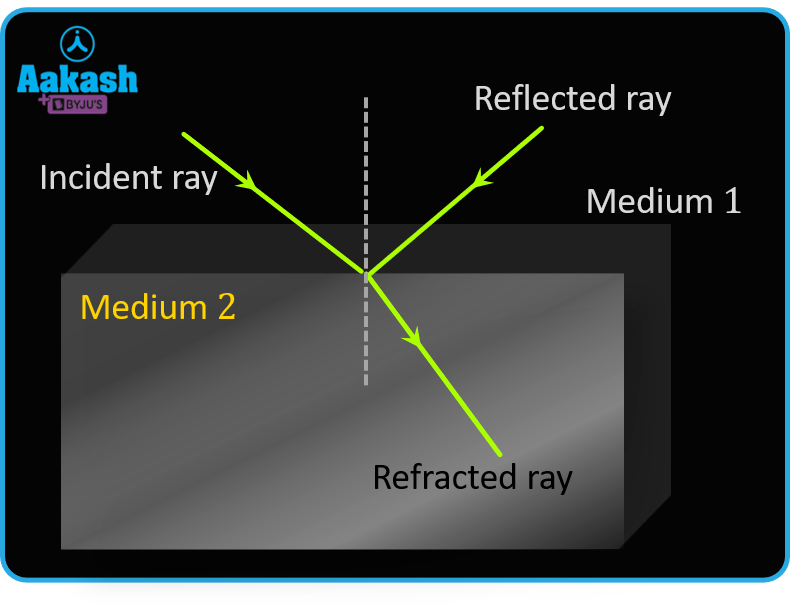## Plane Mirror

A plane mirror is a plane glass plate, one side of which is polished and coated with mercury such that it can reflect most of the light falling on it, and the other side acts as a mirror.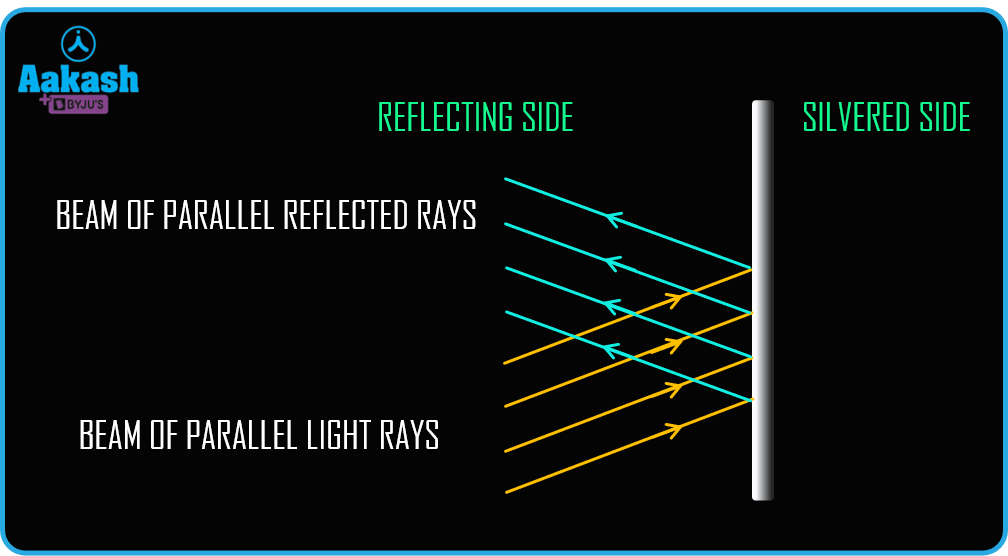When a beam of light (a bunch of photons) is incident on a Plane mirror, the phenomenon of reflection occurs, as shown in the figure.

Object: Object is the point of intersection of incident rays. If the point of intersection is actual, then it will be a real object, and if the point of intersection is apparent, then it will form a virtual object.

Image: Image is the point of intersection of reflected/refracted rays. For this case as well, the actual point of intersection forms a real image, and the apparent point of intersection forms a virtual image.

Now, consider a diverging incident beam that falls on the plane mirror. Here the intersection of the incident beam is in front of the mirror and the intersection of the reflected beam is at the silvered side of the mirror. Hence, for this case, the object will be ‘real’ but the image will be ‘virtual’ as shown in the figure.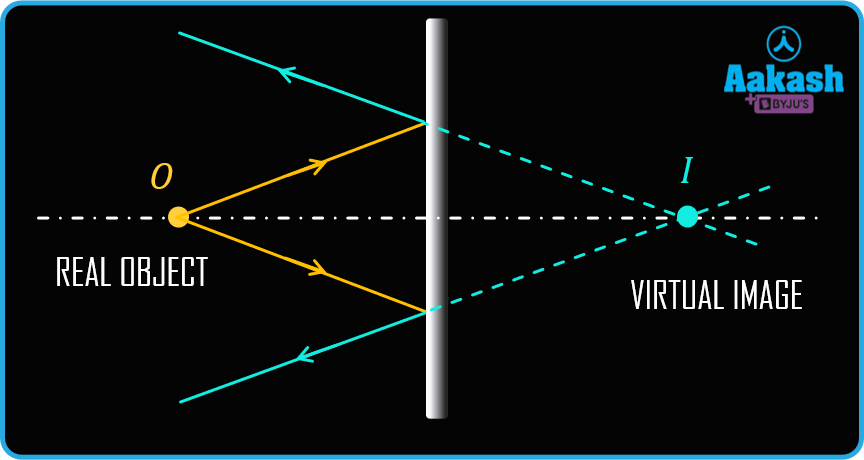Consider a non-intersecting converging beam falling on a plane mirror. Before the reflection from the plane mirror, beams seem to converge and intersect behind the mirror. Hence, the intersection point is known as the virtual object. The reflected beams converge and intersect at a point in front of the mirror. This point can be projected on a screen and therefore, this point of intersection of reflected rays is known as the real image.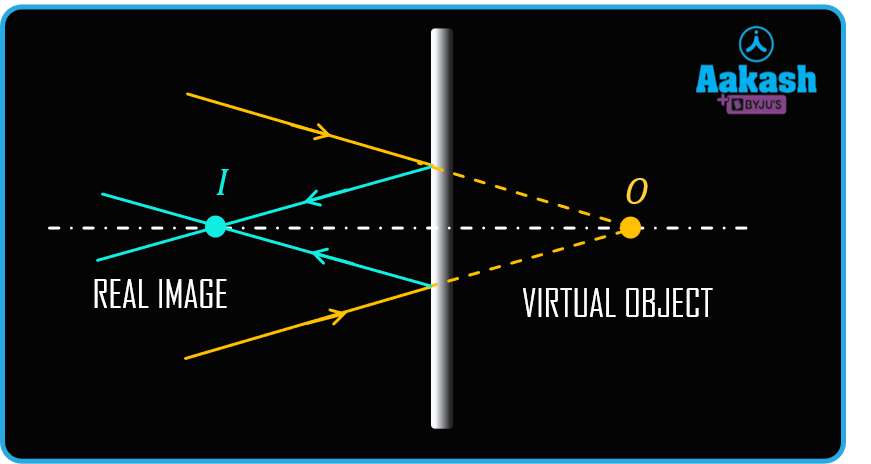## Laws of Reflection

The light ray is reflected from a plane surface and obeys the following two laws of reflection:

1. The angle of incidence is equal to the angle of reflection2. The incident ray, the reflected ray, and the normal to the reflecting surface at the point of incidence are coplanar.
3.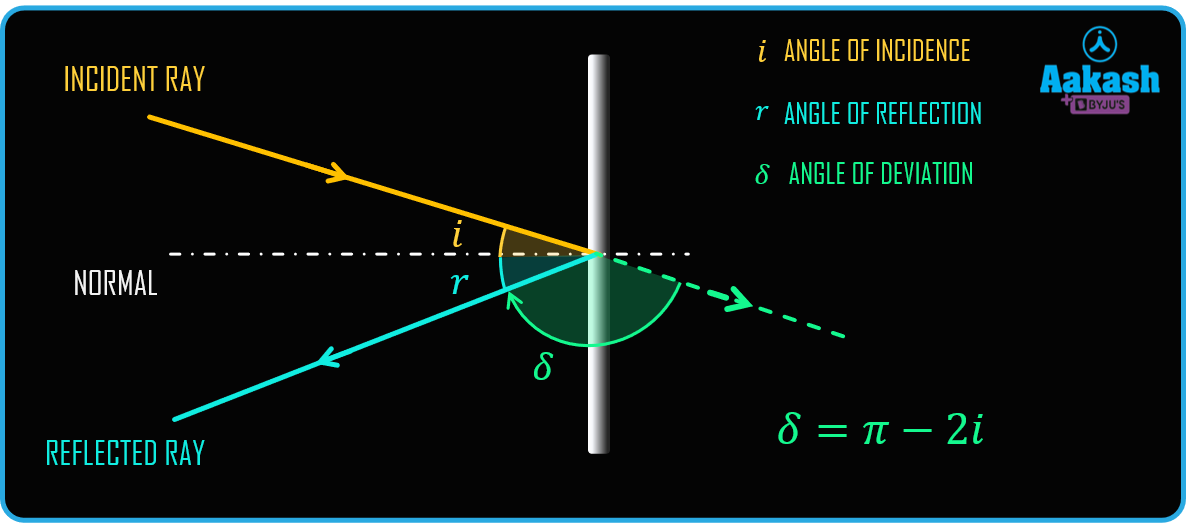A few terminologies regarding reflection are the following:

• Angle of incidence: The angle that the incident ray makes with the normal is known as the angle of incidence, and it is denoted by i.
• Angle of reflection: The angle that the reflected ray makes with the normal is known as angle of reflection, and it is denoted by r.
• Angle of deviation: The angle of deviation is the angle between the direction of the reflected ray and the direction of incident ray in the absence of the interface, and it is denoted by δ.

Now from the figure, it is evident that,From the first law of reflection, i = r

Therefore## Reflection of a Point Object

The rays coming from the object reflect and form a virtual image of the object.

The distance between the image and the mirror is the same as the distance between the object and the mirror.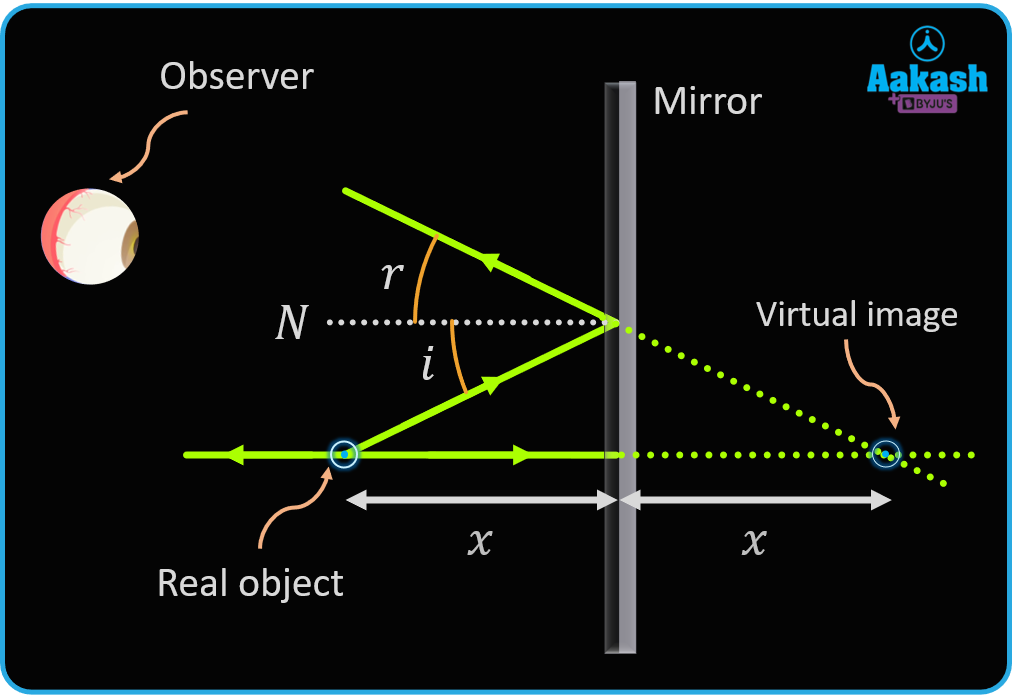## Reflection of an Extended Object

The objects that we see in our daily life, all of them are extended objects. The following are the steps to draw an image of an extended object.

1. Draw a normal incident ray from the topmost (A) and the bottom-most point (B) of the extended object and extend them to the back of the mirror.
2. Now, draw an incident ray from point A that falls at an angle on the mirror and reflects. On extending the line to the back of the mirror, it meets at point A′. This is the image of point A.
3. Do the same for point B and let the point where both the lines meet be B′.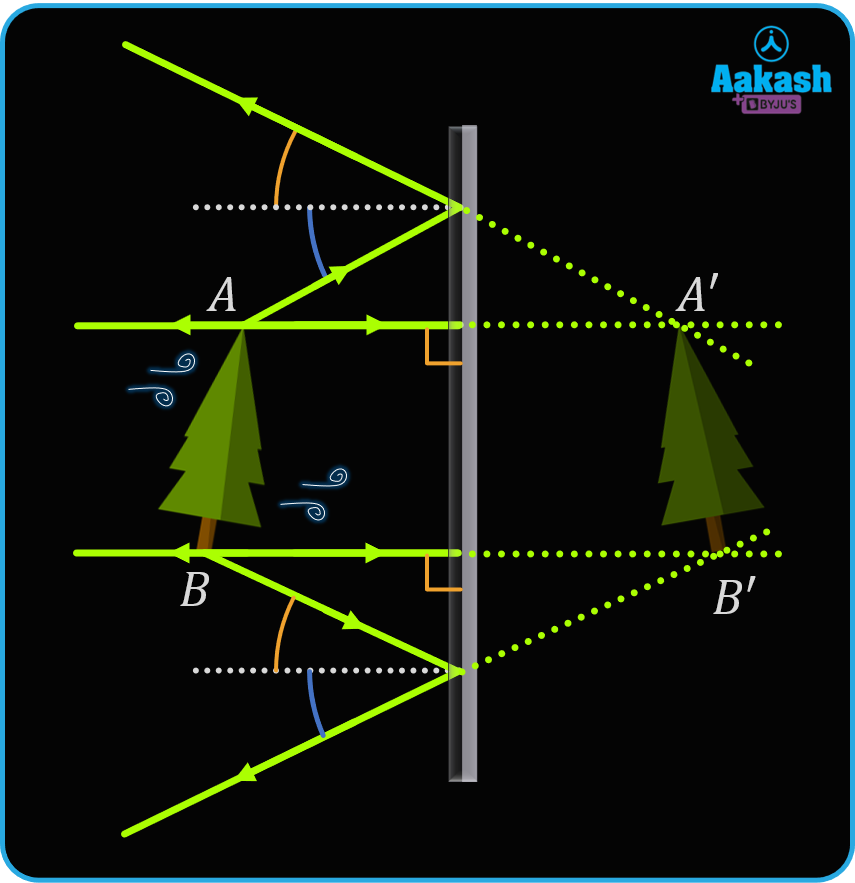The properties of an image formed by plane mirrors are as follows:

1. Virtual image
2. Upright
3. Laterally inverted
4. Same size
5. Object distance from mirror = image distance from mirror

## Field of View

The field of view is the region in which an observer's eye must be present in order to view the image. An observer, who is not in the field of view, will not be able to see the image. The shaded, conical region in the figure is the field of view.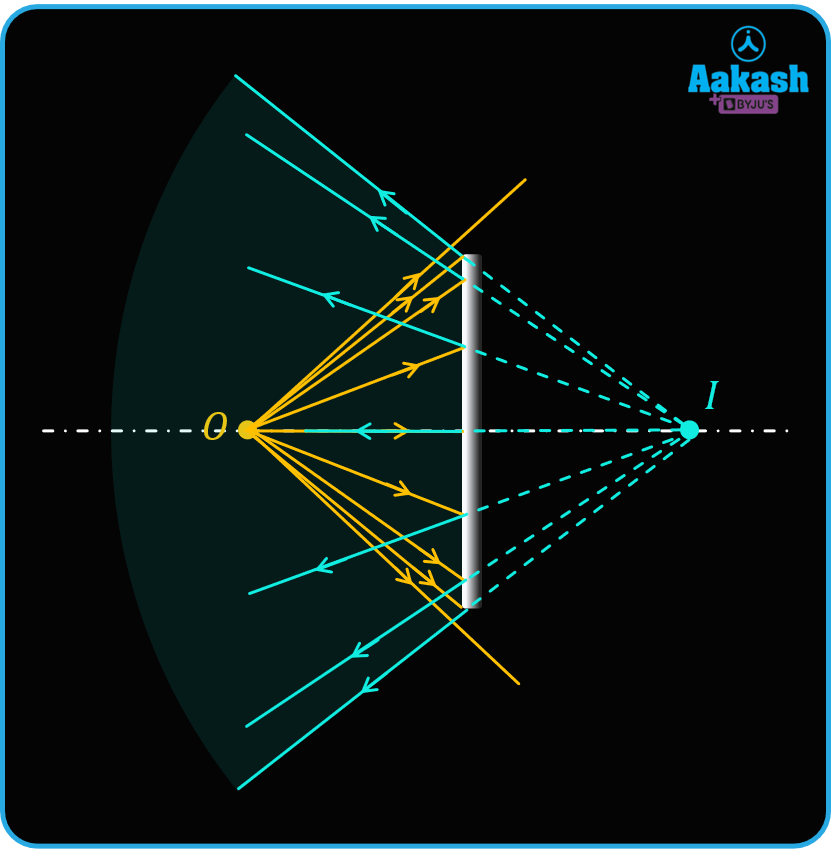## Practice Problems of Optics

Question.1 A ray of light incident on a plane mirror at an angle of 30°. What is the deviation produced by the ray?

1. 30°
2. 60°
3. 90°
4. 120°

Incident angle, i = 30°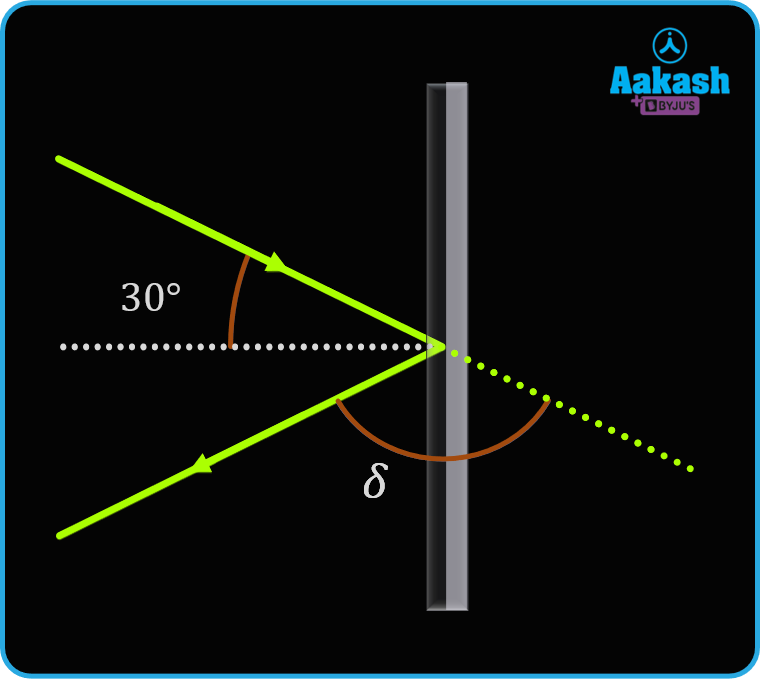Then, angle of deviation is,

⇒ 𝛿 = 180° - 2i

⇒ 𝛿 = 180° - 2(30°)

⇒ 𝛿 = 120°

Thus, option (D) is the correct answer.

Question.2 .A man with a height of 6 ft wants to see his full image. What will be the minimum length of the mirror?

1. 12 ft
2. 3 ft
3. 6 ft
4. 18 ft

Answer.For the man to observe his full image in the mirror, we have to take two parts.

First is the light ray from his head coming to his eyes, and the second is the light ray coming from the toe of the man to his eye, as shown in the given figure.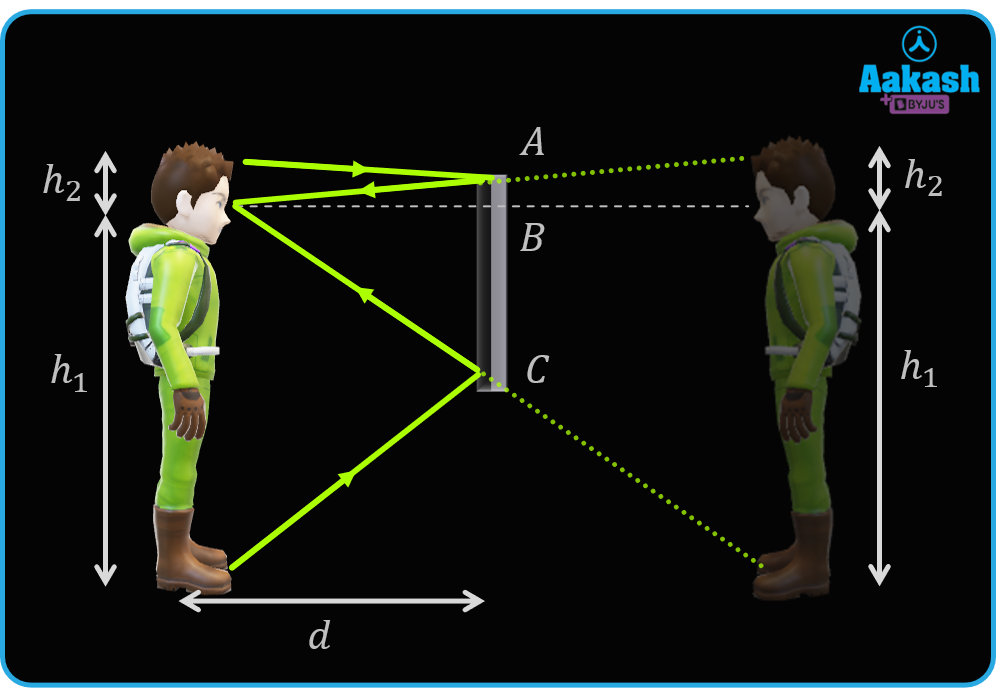For the first part, we get,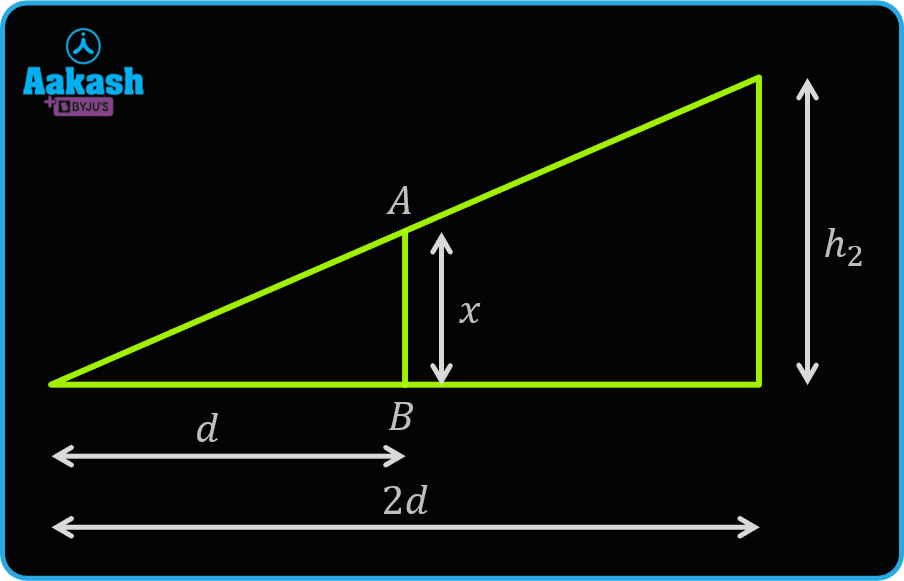By using the concept of similar triangle, we get,For the second part, we get,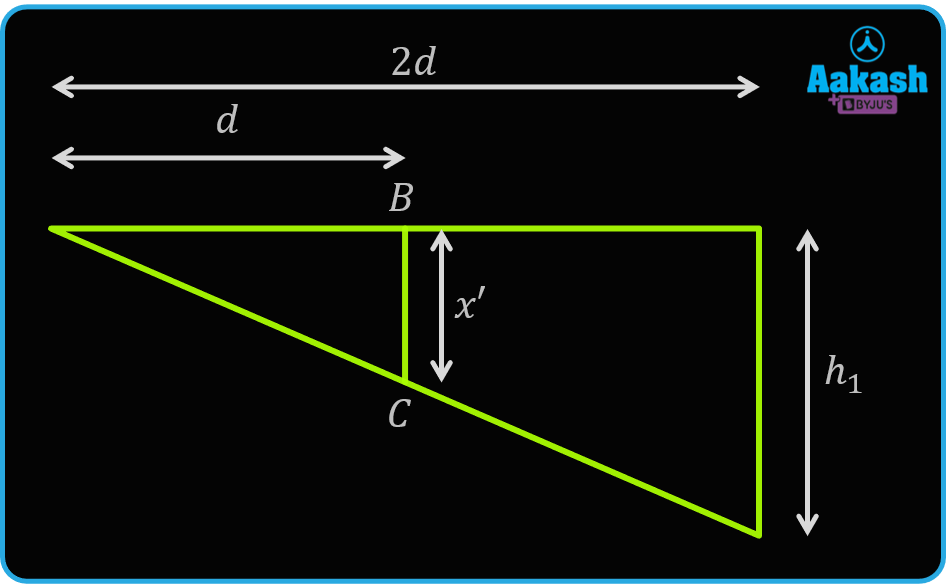By using the concept of similar triangle, we get,The length of mirror,Thus, option (B) is the correct answer.

Question.3  A man is traveling on a long road with a point object O placed right between the road and a mirror of length l. Find the length of the road for which the image of the object is visible to him.

1. l
2. 3l
3. 1.5l
4. 2l

The length of the mirror is l.

Let the length of the field of view of the object on the road be 2x.

From the ray diagram, we get,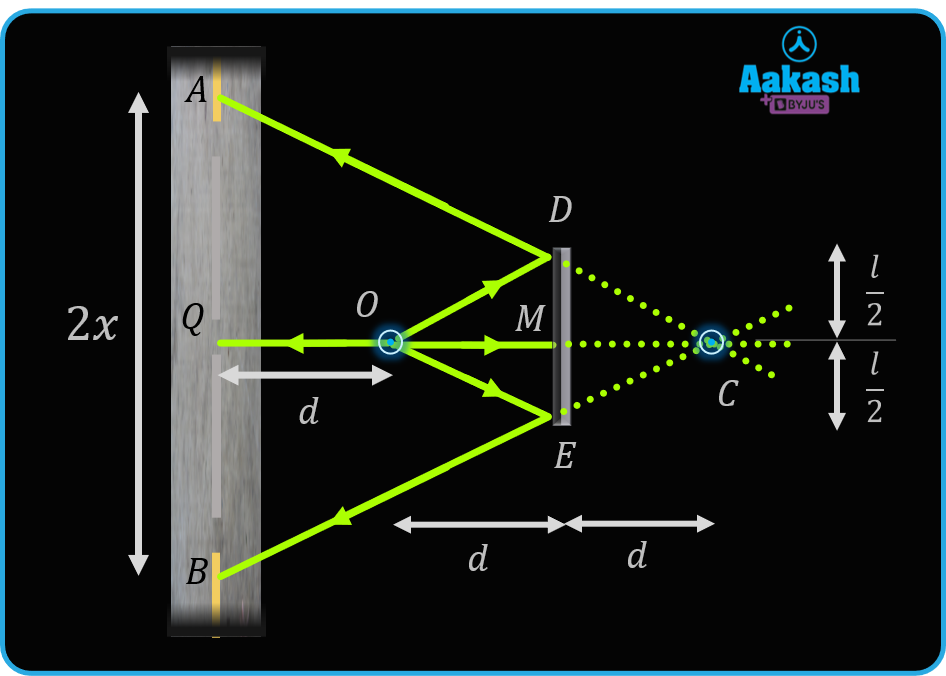By using the concept of similar triangle, we get,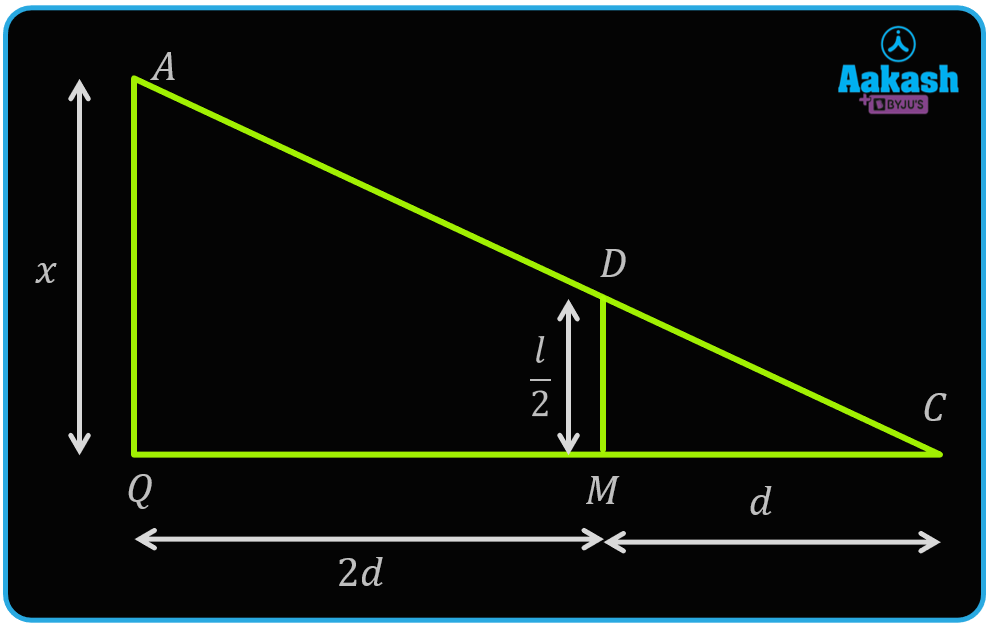Thus, option (B) is the correct answer.

Question.4 The figure shows a point object (A) and a plane mirror (MN). Find the position of the image of object A in mirror MN by drawing ray diagram. Indicate the region in which the observer's eye must be present in order to view the image.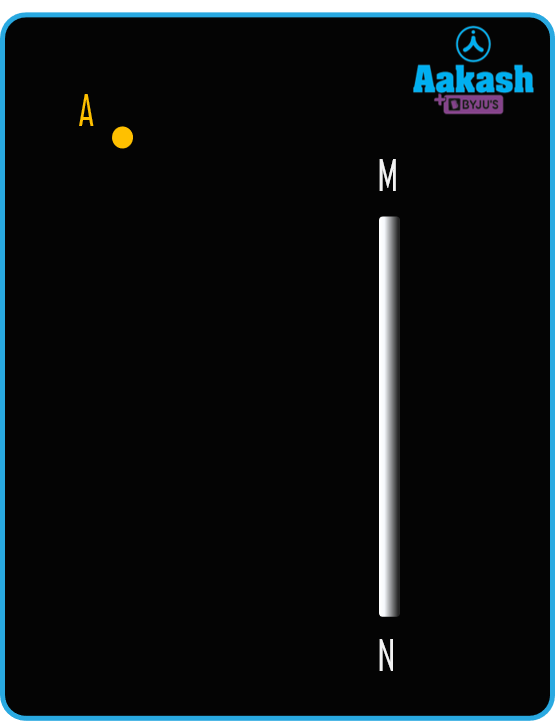Answer.We know that the plane mirror is the perpendicular bisector of a line joining the point object and point image. Using the following ray diagram, we can obtain the same.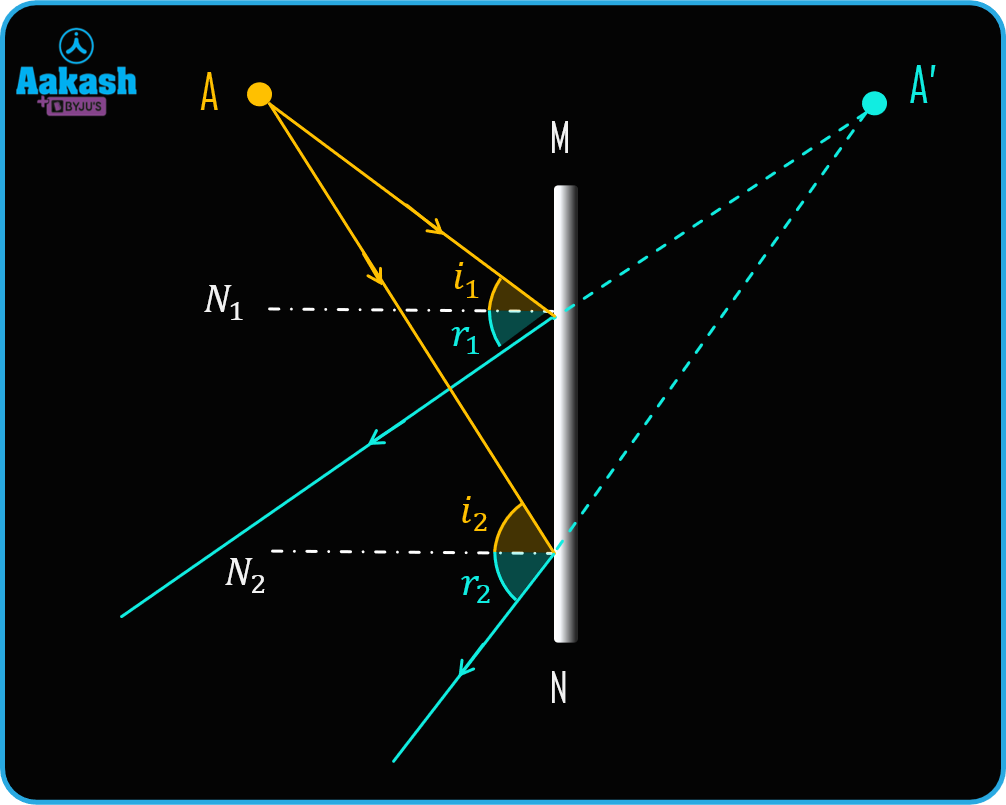Now, we will draw the reflected ray from two extreme positions of the plane mirror as shown in the figure. The region between those two reflected rays from the extremities is known as the field of view.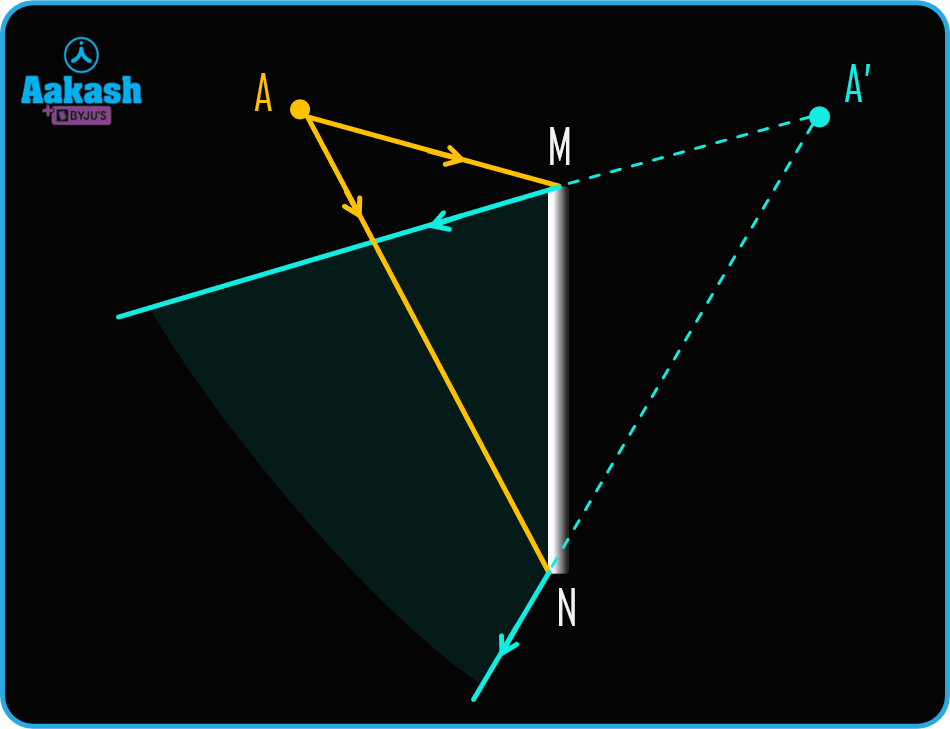An observer's eye must be present in the shaded region in the figure in order to view the image.

## FAQs of Optics

Question.1 What are the various instruments in optics?

Answer. Optical instruments are mirrors, lenses, telescopes, microscopes, lasers, optical fiber etc.

Question.2  What is the size of an image in a plane mirror?

Answer. Size of the image is the same as the object size.

Question.3 Who is the father of optics?

Answer. Ibn al-Haitham is known as the father of optics.

Question.4 What are the various laws of optics?

Answer. Two basic laws of optics are-

1. Law of reflection
2. Law of refraction or snell’s law

## Related Topics to Optics in Physics

• Introduction to optics and Plane mirrors
• Image formation due to parallel and perpendicular mirrors, rotation of plane mirror
• Image formation due to inclined plane mirrors
• Velocity of image in plane mirror
• Convex lens
• Concave lens
• Human Eye
• Simple Microscopes
• Compound microscope
• Telescopes
• Mirror Formula

## NCERT Class 12 Physics Chapters

 Electric Charges And Fields Electrostatic Potential and Capacitance Current Electricity Moving Charges and Magnetism Magnetism and Matter Electromagnetic Induction Alternating Current Electromagnetic Waves Ray Optics and Optical Instruments Wave Optics Dual Nature of Radiation And Matter Atoms Nuclei Semiconductor Electronics Communication Systems
Talk to our expert
By submitting up, I agree to receive all the Whatsapp communication on my registered number and Aakash terms and conditions and privacy policy